# CLASS 10 MATH NCERT SOLUTIONS FOR CHAPTER – 6 TRIANGLES EX – 6.5

## Triangles

Question 1.
Sides of triangles are given below. Determine which of them are right triangles. In case of a right triangle, write the length of its hypotenuse.

(i) 7 cm, 24 cm, 25 cm
(ii) 3 cm, 8 cm, 6 cm
(iii) 50 cm, 80 cm, 100 cm
(iv)
13 cm, 12 cm, 5 cm

Solution: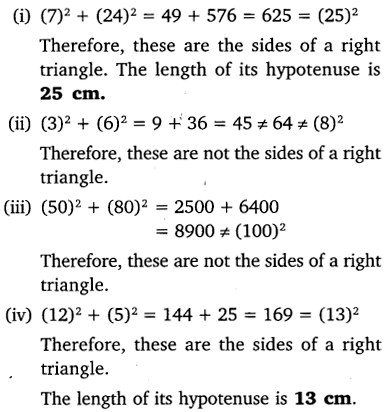Question 2.
PQR is a triangle right angled at P and M is a point on QR such that PM ⊥ QR. Show that PM2 = QM X MR.

Solution:
In a right triangle, perpendicular drawn from right angle to hypotenuse divides the triangle into two similar triangles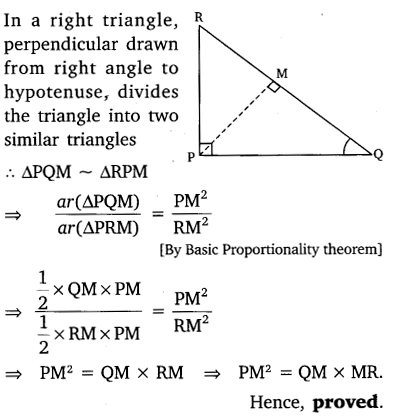Question 3.
In the given figure, ABD is a triangle right angled at A and AC i. BD. Show that

(i) AB2 = BC.BD
(ii) AC2 = BC.DC
(iii) AD2 = BD.CD

Solution: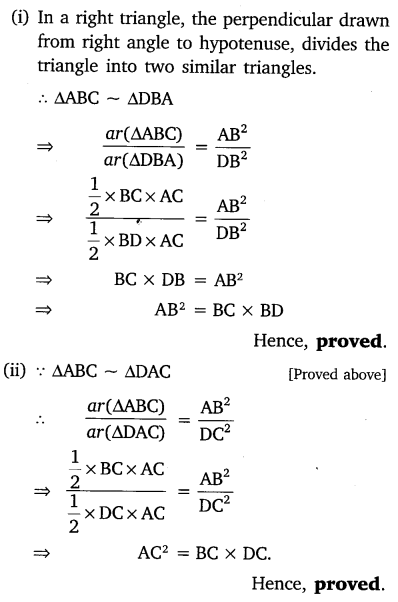Question 4.
ABC is an isosceles triangle right angled at C. Prove that AB2 = 2AC2.
Solution: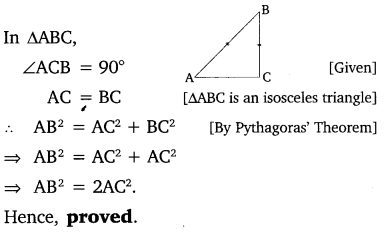Question 5.
ABC is an isosceles triangle with AC = BC. If AB2 = 2AC2, Prove that ABC is a right triangle.

Solution: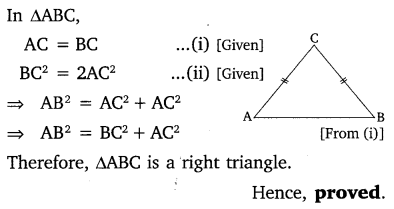Question 6.
ABC is an equilateral triangle of side la. Find each of its altitudes.

Solution: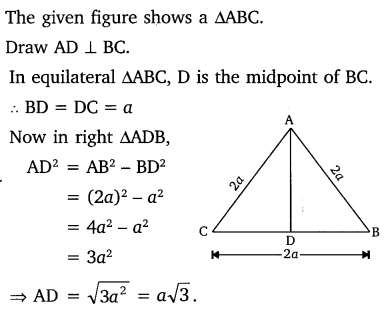As all the altitudes of an equilateral triangle are equal hence, each of the altitudes of ∆ABC is of length$\sqrt { 3 } a$.

Question 7.
Prove that the sum of the squares of the sides of a rhombus is equal to the sum of the squares of its diagonals.

Solution:
The figure given below shows a rhombus ABCD in which AB = BC = CD = DA. The diagonals AC and BD bisect each other at O.
In ∆AOB, ∠AOB = 90°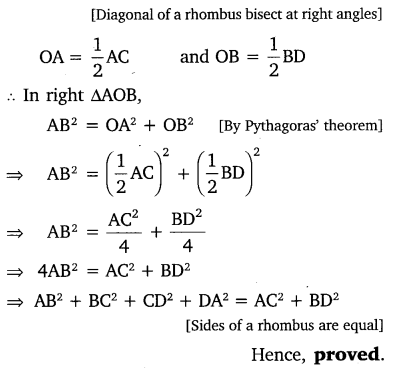Question 8.
In the given figure, O is a point in the interior of a triangle ABC, OD ⊥ BC, OE ⊥ AC and OF ⊥ AB. Show that

(i) OA2 + OB2 + OC2 – OD2 – OE2 – OF2 = AF2 + BD2 + CE2
(ii) AF2 + BD2 + CE2 = AE2 + CD2 + BF2.

Solution: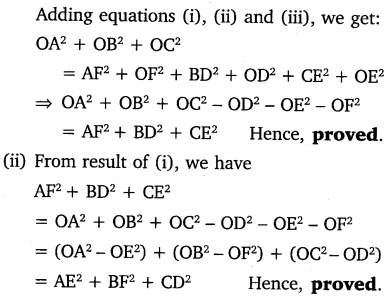Question 9.
A ladder 10 m long reaches a window 8 m above the ground. ind the distance of the foot of the ladder from base of the wall.

Solution: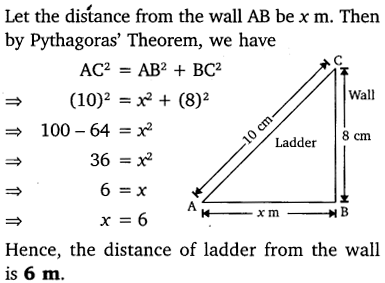Question 10.
A guy wire attached to a vertical pole of height 18 m is 24 m long and has a stake attached to the other end. How far from the base of the pole should the stake be driven so that the wire will be taut?

Solution: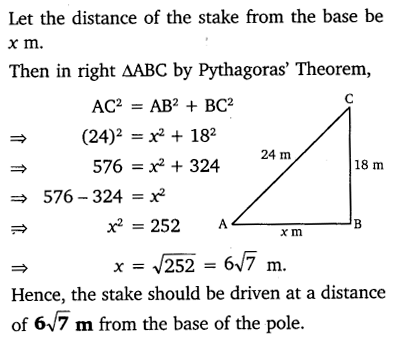Question 11.
An airplane leaves an airport and flies due north at a speed of 1000 km per hour. At the same time, another airplane leaves the same airport and flies due west at a speed of 1200 km per hour. How far apart will be the two planes after 1 hours?

Solution:Question 12.
Two poles of heights 6 m and 11m stand on a plane ground. If the distance between the feet of the poles is 12 m, find the distance between their tops.

Solution: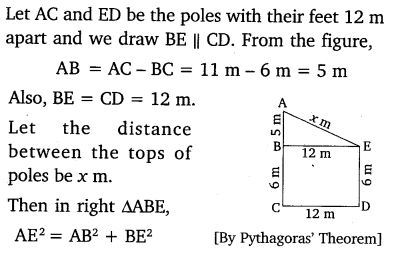Question 13.
D and E are points on the sides CA and CB respectively of a triangle ABC right angled at C. Prove that AE2 + BD2 = AB2 + DE2.

Solution: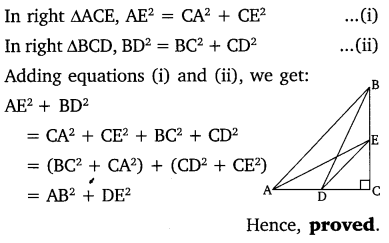Question 14.
The perpendicular from A on side BC of a ∆ABC intersects BC at D such that DB = 3CD (see the figure). Prove that 2AB= 2AC2 + BC2.

Solution: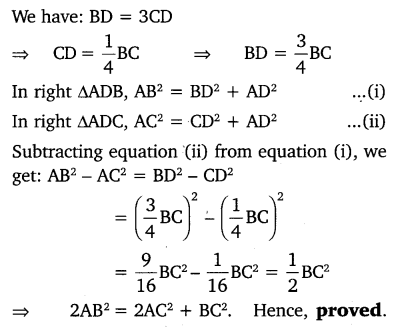Question 15.
In an equilateral triangle ABC, D is a point on side BC, such that BD = BC. Prove that 9AD2 = 7AB2.

Solution: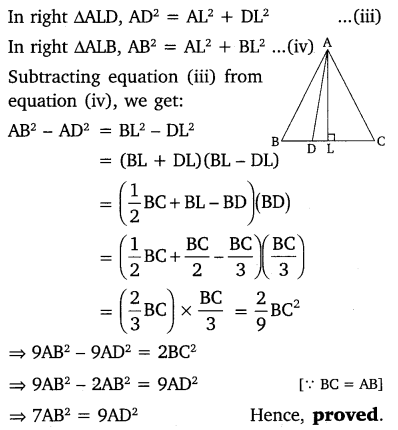Question 16.
In an equilateral triangle, prove that three times the square of one side is equal to four times the square of one of its altitudes.

Solution: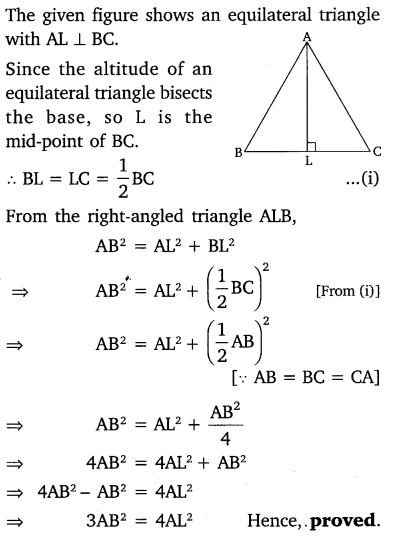Question 17.
Tick the correct answer and justify : In ∆ABC, AB = 6cm, AC = 12 cm and BC = 6 cm. The angle B is:
(a) 120°
(b) 60°
(c) 90°
(d) 45

Solution: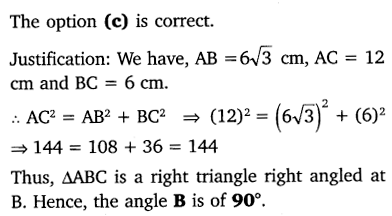### Take Your IIT JEE Coaching to Next Level with Examtube

• Mentoring & Teaching by IITians
• Regular Testing & Analysis
• Preparation for Various Engineering Entrance Exams
• Support for School/Board Exams
• 24/7 Doubts Clarification